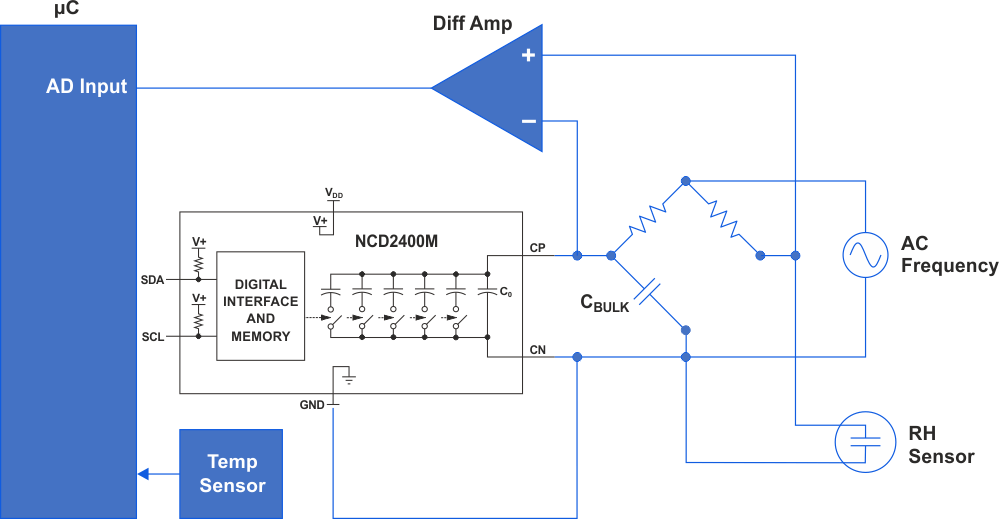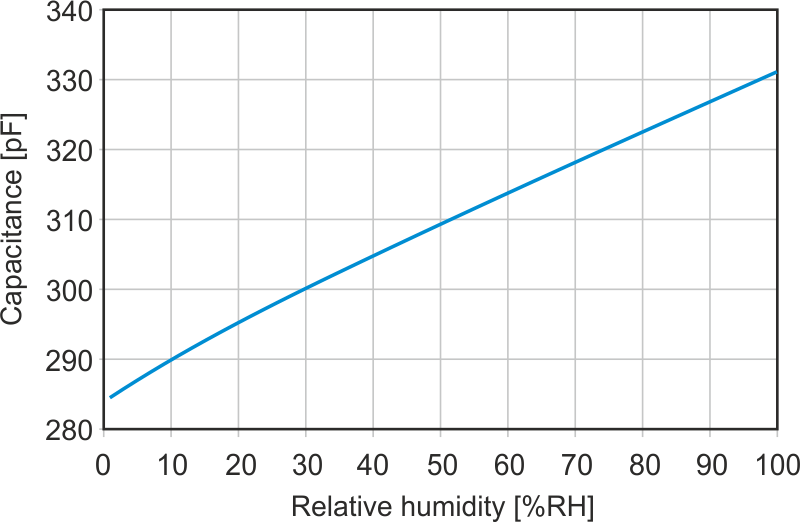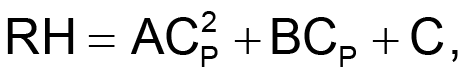# Measure humidity with an RH bridge and digitally-programmable capacitor

## IXYS NCD2400M

Michael Gambuzza

EDN

An AC bridge circuit can be used to measure the unknown capacitance of a sensor, such as a relative humidity (RH) sensor. Some RH sensors have a transfer function of capacitance vs %RH. Usually the value of the capacitance change (∆C) from 0% to 100% is quite small. Using the NCD2400 digitally programmable capacitor array with high step resolution can help determine the value of the unknown capacitance and the RH using a standard AC bridge circuit.

The NCD2400 is an I2C controlled device in a tiny DFN package that offers a wide capacitance range and non-volatile operation with 512-state digital capacitor states. Data is written to it over a standard I2C 2-wire bus. Figure 1 shows the typical circuit.Figure 1. Using the NCD2400 digitally programmable capacitor array with high step resolution can help determine the value of the unknown capacitance and the RH using a standard AC bridge circuit.

The circuit functions as a simple AC bridge circuit with matching resistor values and a stable fixed frequency source. CBULK can be fixed at the 0% RH capacitance of the particular RH sensor that will be used. For example, in Figure 2, the 0% bulk capacitance is about 270 pF. Setting a fixed capacitor in the bridge of this approximate value allows the NCD shunt capacitance to be swept from 12.5 pF to 60 pF in 355 fF steps (133 discrete values in this example). This swept capacitance correlates to a span of 282.5 pF to 330 pF or 0% to 100% RH based on the graph in Figure 2.Figure 2. The 0% bulk capacitance is about 270 pF.

As capacitance is swept by writing to the NCD2400 from low capacitance to high capacitance, the differential amplifier outputs the difference voltage in the bridge. When the voltage minima is detected by the ADC/µC, the capacitance value of the NCD matches the RH sensor capacitance. At this point the capacitor value can be used to determine the RH%.

## Calibration

Calibration of the system is critical, and the system is usually calibrated in a humidity chamber to determine the calibration curve of the sensor. Since the relationship between RH and capacitance is not necessarily linear, the calibration will more likely yield a quadratic transfer function in the form:where CP = sensor capacitance and A, B, C are coefficients.

Replacing CP transfer function with the capacitance value obtained using the NCD and nulling the circuit will yield the RH result. The RH result, along with the measured temperature value from the temperature sensor in Figure 1, is then used to calculate the temperature compensated RH result as this will yield the most accuracy.

RH measurement is a complex task and all measurement uncertainties should be taken into account. Fortunately, if calibration is performed at the system level, many of the stray capacitances and branch impedances cancel and repeatability is good.

Using the NCD2400 digitally programmable capacitor array with high step resolution can help determine the value of the unknown capacitance and the RH using a standard AC bridge circuit.

EDN